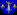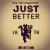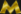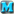<< Back to Strategy Forum .GrayedOutTextBox { color: Gray; }

 Posts 1 - 2 of 2
Luck Mod Math question: 5/15/2017 17:34:44Andy the Nerdherder
Level 58
Report
Does anyone know if the math on luck rounds the "expected kills" number before it averages it with the luck kills?

Example:

8 defenders, 70% kill rate: Expected kills would be 5.6, and would (straight) round to 6.

If you have 50% luck, and it rolled 7 kills (always an integer), do you get 5.6 * 50% + 7 * 50% = 2.8 + 3.5 = 6.3 which (straight) rounds to 6, or is it 6 * 50% + 7 * 50% = 3 + 3.5 = 6.5 which (straight) rounds to 7 which kills a commander...

If the expected kills get rounded before the luck numbers get factored in, does the rounding mode (weighted / straight) also get used in that rounding calc?

Anywho, all the examples I found in the wiki or the forum have integer expectations (10 armies with 70%) making my question moot.

Cheers,
Luck Mod Math question: 5/15/2017 17:55:27DeadmanLevel 64
Report
This is my understanding of how it works. Fizzer or someone else will correct me if I'm wrong.

8 defenders, 70% kill rate: Expected kills would be 5.6, and would (straight) round to 6.

If you have 50% luck, and it rolled 7 kills (always an integer), do you get 5.6 * 50% + 7 * 50% = 2.8 + 3.5 = 6.3 which (straight) rounds to 6, or is it 6 * 50% + 7 * 50% = 3 + 3.5 = 6.5 which (straight) rounds to 7 which kills a commander...

If you have 50% luck and it rolled 7 kills, the number of armies killed is the weighted mean (50% of 5.6 + 50% of 7 = 2.8+3.5 = 6.3).

If it was 60% luck and it rolled 7 kills, the number of armies killed would be 40% of 5.6 + 60% of 7 = 2.24 + 4.2 = 6.44.

After it has determined how many armies should be killed, the rounding mode determines how a decimal is rounded. That is, SR would round 6.3(or 6.44) to 6.

WR would round 6.3 to 6(70% of the time) and round to 7 (30% of the time).
WR would round 6.44 to 6(56% of the time) and round to 7 (44% of the time).
 Posts 1 - 2 of 2